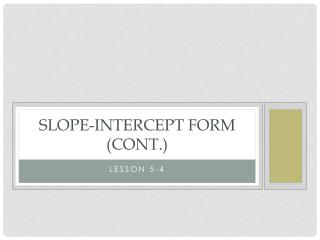DownloadDownload PresentationSlope-Intercept Form (cont.)

# Slope-Intercept Form (cont.)

Télécharger la présentation## Slope-Intercept Form (cont.)

- - - - - - - - - - - - - - - - - - - - - - - - - - - E N D - - - - - - - - - - - - - - - - - - - - - - - - - - -
##### Presentation Transcript

1. Slope-Intercept Form (cont.) Lesson 5-4

2. Slope Review What we’ve learned so far… slope = m rise run slope formula =y² - y¹ x² - x¹ y-intercept = b slope-intercept form  y = mx + b

3. Slope Review Count slope… m = Plot slope… (-1, -3) m = ¾

4. Slope Review Identify & Graph slope-intercept form: y = mx + b y = -2x + 4 m = b =

5. More with slope-intercept form Rewrite an equation to slope-intercept form (SIF): y – 4 = x y = mx + b y – 4 = 3x + 4 +4 y = 3x + 4 m = b =

6. More with slope-intercept form Write in SIF: 2x+ 3y = -9 Write in SIF: y + ¾x = 0

7. More with slope-intercept form Given m & b, write an equation in slope-intercept form (SIF): m = - ½, b = -5  y = mx + b plug in… y = -½ x - 5

8. More with slope-intercept form Write in SIF: m = 2/3, b = 0 Plug in… y = mx + b Write in SIF: m = 0, b = -2 Plug in… y = mx + b

9. Practice Write the following in Slope Intercept Form: • 2x – y = 7 • 4y + 3x = -8 • m = -1/3, b = 5 • m = 2, b = -1

10. homework p. 233-234 #14-16, 27-29, 31-33, 35-38